1593153745

# Specific Explanation Multivariate Linear Regression in Python

Linear regression is probably the most simple machine learning algorithm. It is very good for starters because it uses simple formulas. So, it is good for learning machine-learning concepts. In this article, I will try to explain the multivariate linear regression step by step.

## Concepts and Formulas

Linear regression uses the simple formula that we all learned in school:

Y = C + AX

Just as a reminder, Y is the output or dependent variable, X is the input or the independent variable, A is the slope, and C is the intercept.

For the linear regression, we follow these notations for the same formula: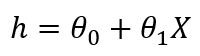If we have multiple independent variables, the formula for linear regression will look like: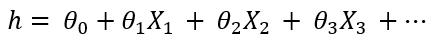Here, ‘h’ is called the hypothesis. This is the predicted output variable. Theta0 is the bias term and all the other theta values are coefficients. They are initiated randomly in the beginning, then optimized with the algorithm so that this formula can predict the dependent variable closely.

## Cost Function and Gradient Descent

When theta values are initiated in the beginning, the formula is not trained to predict the dependent variable. The hypothesis is far away from the original output variable ‘Y’. This is the formula to estimate the cumulative distance of all the training data: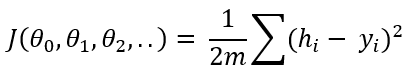This is called the cost function. If you notice, it deducts y(the original output) from the hypothesis(the predicted output), takes the square to omit the negativity, sum up and divide by 2 times m. Here, m is the number of training data. You probably can see that cost function is the indication of the difference between the original output and the predicted output. The idea of a machine learning algorithm is to minimize the cost function so that the difference between the original output and the predicted output is closer. To achieve just that, we need to optimize the theta values.

Here is how we update the theta values. We take the partial differential of the cost function with respect to each theta value and deduct that value from the existing theta value,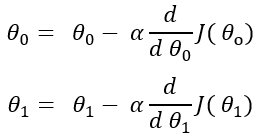Here, alpha is the learning rate and it is a constant. I am not showing the same formula for all the theta values. But It is the same formula for all the theta values. After the differentiation, the formula comes out to be: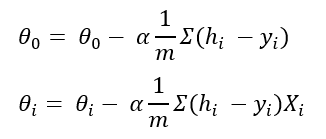## Implementation of the Algorithm Step by Step

The dataset I am going to use is from Andre Ng’s machine learning course in Coursera. I will provide the link at the bottom of this page. Please feel free to download the dataset and practice with this tutorial. I encourage you to practice with the dataset as you read if this is new to you. That is the only way to understand it well.

In this dataset, there are only two variables. But I developed the algorithm for any number of variables. If you use the same algorithm for 10 variables or 20 variables, it should work as well. I will use Numpy and Pandas library in Python. All these rich libraries of Python made the machine learning algorithm a lot easier. Import the packages and the dataset:

``````import pandas as pd
import numpy as np

``````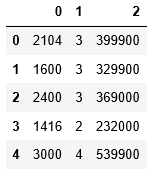#machine-learning #data-science #machine-intelligence #python

## Buddha Community1619518440

## top 30 Python Tips and Tricks for Beginners

Welcome to my Blog , In this article, you are going to learn the top 10 python tips and tricks.

### 8) Check The Memory Usage Of An Object.

#python #python hacks tricks #python learning tips #python programming tricks #python tips #python tips and tricks #python tips and tricks advanced #python tips and tricks for beginners #python tips tricks and techniques #python tutorial #tips and tricks in python #tips to learn python #top 30 python tips and tricks for beginners1592023980

## 5 Regression algorithms: Explanation & Implementation in Python

Take your current understanding and skills on machine learning algorithms to the next level with this article. What is regression analysis in simple words? How is it applied in practice for real-world problems? And what is the possible snippet of codes in Python you can use for implementation regression algorithms for various objectives? Let’s forget about boring learning stuff and talk about science and the way it works.

#linear-regression-python #linear-regression #multivariate-regression #regression #python-programming1619510796

## Lambda, Map, Filter functions in python

Welcome to my Blog, In this article, we will learn python lambda function, Map function, and filter function.

Lambda function in python: Lambda is a one line anonymous function and lambda takes any number of arguments but can only have one expression and python lambda syntax is

Syntax: x = lambda arguments : expression

Now i will show you some python lambda function examples:

#python #anonymous function python #filter function in python #lambda #lambda python 3 #map python #python filter #python filter lambda #python lambda #python lambda examples #python map1594271340

## A Deep Dive into Linear Regression

Let’s begin our journey with the truth — machines never learn. What a typical machine learning algorithm does is find a mathematical equation that, when applied to a given set of training data, produces a prediction that is very close to the actual output.

Why is this not learning? Because if you change the training data or environment even slightly, the algorithm will go haywire! Not how learning works in humans. If you learned to play a video game by looking straight at the screen, you would still be a good player if the screen is slightly tilted by someone, which would not be the case in ML algorithms.

However, most of the algorithms are so complex and intimidating that it gives our mere human intelligence the feel of actual learning, effectively hiding the underlying math within. There goes a dictum that if you can implement the algorithm, you know the algorithm. This saying is lost in the dense jungle of libraries and inbuilt modules which programming languages provide, reducing us to regular programmers calling an API and strengthening further this notion of a black box. Our quest will be to unravel the mysteries of this so-called ‘black box’ which magically produces accurate predictions, detects objects, diagnoses diseases and claims to surpass human intelligence one day.

We will start with one of the not-so-complex and easy to visualize algorithm in the ML paradigm — Linear Regression. The article is divided into the following sections:

1. Need for Linear Regression

2. Visualizing Linear Regression

3. Deriving the formula for weight matrix W

4. Using the formula and performing linear regression on a real world data set

Note: Knowledge on Linear Algebra, a little bit of Calculus and Matrices are a prerequisite to understanding this article

Also, a basic understanding of python, NumPy, and Matplotlib are a must.

## 1) Need for Linear regression

Regression means predicting a real valued number from a given set of input variables. Eg. Predicting temperature based on month of the year, humidity, altitude above sea level, etc. Linear Regression would therefore mean predicting a real valued number that follows a linear trend. Linear regression is the first line of attack to discover correlations in our data.

Now, the first thing that comes to our mind when we hear the word linear is, a line.

Yes! In linear regression, we try to fit a line that best generalizes all the data points in the data set. By generalizing, we mean we try to fit a line that passes very close to all the data points.

But how do we ensure that this happens? To understand this, let’s visualize a 1-D Linear Regression. This is also called as Simple Linear Regression

#calculus #machine-learning #linear-regression-math #linear-regression #linear-regression-python #python1622168430

## Implementing Simple Linear Regression in Python from Scratch

Today we will implement one of the most famous machine learning algorithms, Simple Linear Regression from scratch and then with scikit-learn module and then compare the two models. The code for this can be found on this Github Link.

## Conclusion

#linear-regression-python #linear-regression #data-science #python #machine-learning